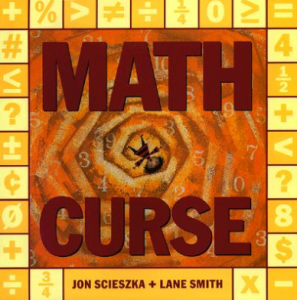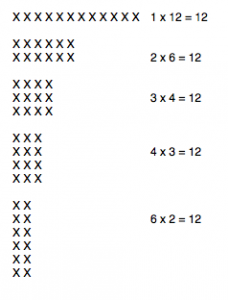# Math CurseI’ve been reading The Math Curse by John Scieska + Lane Smith to my first grade group and I continue to be amazed at what their young minds can absorb!  If you’re not familiar with the book, here is a link to a video of the book being read.  Here is another link to a condensed version of the book (more in movie form).  I have read this book to all ages and there’s really something for everyone in here…no matter what your math background.  Here are the things I worked on with the 1st graders:

Fibonacci’s Sequence:  1, 1, 2, 3, 5, 8, 13, 21…

• I put the sequence on the board and within a minute, the first graders were able to figure out the pattern.  What are the next 3 numbers in this sequence?  What would the first 10 terms in the sequence be if Fibonacci’s sequence started with 2 fours?

Gallons-Quarts-Pints-Cups

• The graphic below is often used to teach volume conversions.  There are 4 quarts in a gallon, 2 pints in a quart, and 2 cups in a pint.  Having this visual makes it easy to answer other conversion questions.  How many pints are in a gallon?  How many cups in a gallon?  How many quarts are in a half gallon?Arrays

• Instead of 24 kids in the class, the first graders helped figure out seating arrangements for 12 kids.  They easily came up with 1 row of 12, 2 rows of 6, and so on.  That made it really easy to introduce multiplication.  We also had fun counting how many ears, fingers, eyes, etc. in the classroom.  As an extension, I asked questions like: What is the number eyes in the classroom take away the number of noses?  What is the number of fingers in the classroom plus the number of arms?The students’ homework is to make up an everyday math problem so we can create a book similar to The Math Curse.  Leave a comment with a math problem that you made up and it just might make it into our book!